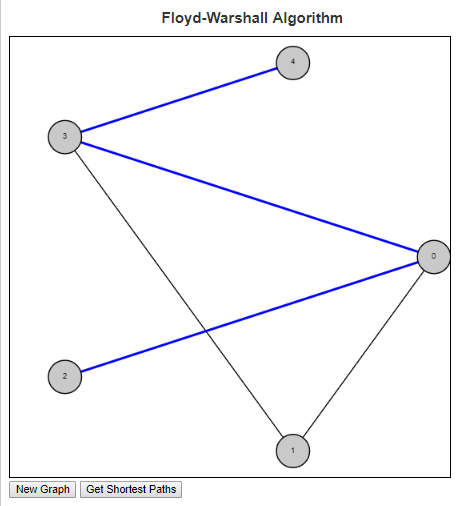# Floyd-Warshall Shortest PathsThe Floyd Warshall algorithm is an all pairs shortest paths algorithm. This can be contrasted with algorithms like Dijkstra’s which give the shortest paths from a single node to all other nodes in the graph.

Floyd Warshall’s algorithm works by considering first the edge set of the graph. This is the set of all paths of the graph through one edge. Node pairs that are connected to one another through an edge will have their shortest path set to the length of that edge, while all other node pairs will have their shortest path set to infinity. The program then runs through every triplet of nodes (i, j, k) and checks if the path from i to k and the path from k to j is shorter than the current path from i to j. If so, then the distance and the path is updated.

So lets consider an example on the graph in the image above. The edge set of this graph is E = {(0, 1), (0, 2), (0, 3), (1, 3), (3, 4)}. So our initial table is:

 0 1 2 3 4 0 inf (0, 1) (0, 2) (0, 3) inf 1 (0, 1) inf inf (1, 3) inf 2 (0, 2) inf inf inf inf 3 (0, 3) (1, 3) inf inf (3, 4) 4 inf inf inf (3, 4) inf

As we look to update the paths, we first look for routes that go through node 0:

Because node 0 connects to both node 1 and node 2, but node 1 does not connect to node 2, we have the following truth holding in the matrix above:
cost(0, 1) + cost(0, 2) < cost(1, 2), so we can update the shortest path from node 1 to node 2 to be (1, 0, 2).

Because node 0 connects to both node 2 and node 3, but node 2 does not connect to node 3, we have the following truth holding in the matrix above:
cost(0, 2) + cost(0, 3) < cost(2, 3), so we can update the shortest path from node 2 to node 3 to be (2, 0, 3).

Because node 3 connects to both node 0 and node 4, but node 0 does not connect to node 4, we have the following truth holding in the matrix above:
cost(0, 3) + cost(3, 4) < cost(0, 4), so we can update the shortest path from node 0 to node 4 to be (0, 3, 4).

Because node 3 connects to both node 1 and node 4, but node 1 does not connect to node 4, we have the following truth holding in the matrix above:
cost(1, 3) + cost(3, 4) < cost(1, 4), so we can update the shortest path from node 1 to node 4 to be (1, 3, 4).

Because node 3 connects to both node 2 and node 4, but node 2 does not connect to node 4, we have the following truth now holding:
cost(2, 3) + cost(3, 4) < cost(2, 4), so we can update the shortest path from node 2 to node 4 to be (2, 0, 3, 4).

The final table giving the list of shortest paths from every node to every other node is given below.

 0 1 2 3 4 0 inf (0, 1) (0, 2) (0, 3) (0, 3, 4) 1 (0, 1) inf (1, 0, 2) (1, 3) (1, 3, 4) 2 (0, 2) (1, 0, 2) inf (2, 0, 3) (2, 0, 3, 4) 3 (0, 3) (1, 3) (2, 0, 3) inf (3, 4) 4 (0, 3, 4) (1, 3, 4) (2, 0, 3, 4) (3, 4) inf

To see more examples and to help answer questions, check out the script in my examples section on the Floyd-Warshall algorithm

## 2 thoughts on “Floyd-Warshall Shortest Paths”

1.Eshika Roy says:

Thank you for posting this helpful blog on the Floyd Warshall shortest path. I can’t wait to try this on my own. Please keep on sharing more helpful tips and tricks in the upcoming posts.

This site uses Akismet to reduce spam. Learn how your comment data is processed.